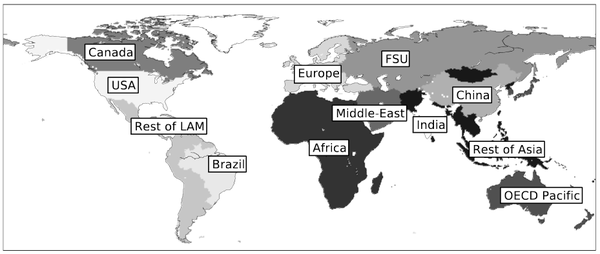# Spatial dimension - IMACLIM

Corresponding documentation
Previous versions
Model information
Institution Centre international de recherche sur l'environnement et le développement (CIRED), France, http://www.centre-cired.fr., Societe de Mathematiques Appliquees et de Sciences Humaines (SMASH), France, http://www.smash.fr.
Solution concept General equilibrium (closed economy)
Solution method SimulationImaclim-R is implemented in Scilab, and uses the fonction fsolve from a shared C++ library to solve the static equilibrium system of non-linear equations.
Anticipation Recursive dynamics: each year the equilibrium is solved (system of non-linear equations), in between two years parameters to the equilibrium evolve according to specified functions.

Imaclim-R is a global model of the world economy, divided into the following 12 regions and shown in Figure 1:

• USA